true
true

Palak K.Tollygunge, Kolkata, India - 700040# Palak K.## Tutor

Tollygunge, Kolkata, India - 700040.

11 Students taught

5.0

UrbanPro Rating
Referral Discount: Get ₹ 500 off when you make a payment to start classes. Get started by Booking a Demo.

Details verified of Palak K.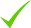IdentityEducation

Know how UrbanPro verifies Tutor details

Identity is verified based on matching the details uploaded by the Tutor with government databases.

## Overview

I have a degree in Mechanical Engineering. I have 11 years of online tutoring experience on Math. Up to class 12. I am fully conversant with Algebra, Trigonometry, Precalculus, Calculus and Descriptive Statistics. I have a passion for Math. During a session, I assess the prior knowledge of the student and structure my lesson accordingly. I teach using Skype, Bit paper interactive whiteboard, digital pen, advanced scientific calculator and various on line graph programs. I work out step by step solution together with the student.I have taught about 12000 students online.I can help students to acquire excellent Mathematical skill. I will be able to adjust my time with the requirement of the students.
I will provide practical examples for a clear understanding of subject topics and plan lessons with achievable goals. I teach with skype, Bitpaper whiteboard, scientific calculator and different graph programs.

Intro Video

• Engineering Diploma Tuition , Class 9 Tuition +6

## Languages Spoken

English Proficient

## Education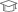Jadavpur University, Kolkata 1965

Bachelor of Engineering (B.E.)

## Address

Tollygunge, Kolkata, India - 700040

## Verified Info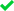ID VerifiedEducation VerifiedPhone VerifiedEmail Verified

Report this Profile

Is this listing inaccurate or duplicate? Any other problem?

Please tell us about the problem and we will fix it.

Please describe the problem that you see in this page.

Type the letters as shown below *

Please enter the letters as show below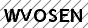Class 10 Tuition

Class LocationOnline Classes (Video Call via UrbanPro LIVE)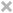Student's HomeTutor's Home

Years of Experience in Class 10 Tuition

15

Board

ICSE, International Baccalaureate, CBSE

IB Subjects taught

Mathematics

CBSE Subjects taught

Mathematics

ICSE Subjects taught

Mathematics

Taught in School or College

No

Teaching Experience in detail in Class 10 Tuition

I am a retired Mechanical engineer. For over 15 years I am doing private tuition out of these 11 years for online tutoring in math. I use Skype,Bitpaper interactive whiteboard, scientific calculator and various graph programs.I explain step by step until the student understands thoroughly.I hold mock tests to prepare the students well before the exams.

## Gallery (2)

Approximation by differentiati...

Application of Trigonometry

## Documents (1)

application of derivative-rate...

## Reviews (3)

this is test message this is test message this is test message this is test message this is test message this is test message this is test message

5.0 out of 5.0 3 reviews

Palak Kanti Maitra https://s3-ap-southeast-1.amazonaws.com/tv-prod/member/photo/5236820-small.png Tollygunge
5.0053
Palak Kanti Maitra
V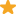"Very good teacher explains all clearly and make sure you have understood everything over all extremely happy with his classes. "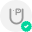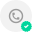Reply by Palak

Thank you madam for appreciating my work.It will definitely inspire me to do better.

Palak Kanti Maitra
S"I am very happy with this teaching. I came to know about many new things which I was not able to understand in the class. I feel proud and blessed to have him as a tutor. He is a great tutor. "

Reply by Palak

Thank you for such a beautiful review,

Palak Kanti Maitra
S"Fantastic and senior professor with clear concepts. Has a lot of patience and can deal with student very well. Very helpful and explains in different ways till the child understands. Sir has through knowledge and very adjusting to the needs of the student. Highly recommend sir. "

Reply by Palak

Thank you so much for writing so wonderful review.

Have you attended any class with Palak? Write a Review

## FAQs

1. Which school boards of Class 10 do you teach for?

ICSE, International Baccalaureate and CBSE

2. Do you have any prior teaching experience?

No

3. Which classes do you teach?

I teach Class 10 Tuition, Class 11 Tuition, Class 12 Tuition, Class 6 Tuition, Class 7 Tuition, Class 8 Tuition, Class 9 Tuition and Engineering Diploma Tuition Classes.

4. Do you provide a demo class?

Yes, I provide a free demo class.

5. How many years of experience do you have?

I have been teaching for 15 years.

## Lessons (7)

Trigonometric ratios for complementary angles

When the sum of two angles=90 degrees, they are called complementary angles. We can use following formula →sin(90-θ)=cosθ,cos(90-θ)=sinθ,tan(90-θ)=cotθ,cosec(90-θ)=secθ,sec(90-θ)=cosecθ We...

05/07/20190
Euclid's divsion lemma application in finding H.C.F.

We know that Euclid's Division lemma states if a and b are two positive integers and a>b, then a=bq+r where q and r are two integers, where the value of r is given by 0<=r<b, This principle is...

22/06/20190
Cube root by estimation

To find cube root by estimation we divide a number into two groups and find ones and tens from each group. Example1):-Estimate cube root of 12167 Here we divide 12167 into two groups 12 and 167 Take...

15/06/20190

## Answers by Palak K. (36)

Answered on 18/07/2019 CBSE/Class 10/Mathematics/UNIT I: Number systems/Real numbers/NCERT Solutions/Exercise 1.1

An army contingent of 616 members is to march behind an army band of 32 members in a parade. The two... ...more
An army contingent of 616 members is to march behind an army band of 32 members in a parade. The two groups are to march in the same number of columns. What is the maximum number of columns in which they can march?

Here a maximum number of columns=H.C.F. between 32 and 616. By division method 616=32x19+8 32=8x4+0, so 8 is H.C, F. between616 and 32.Hence the maximum number of columns=8
Answers 2 Comments
Dislike Bookmark

Answered on 18/07/2019 CBSE/Class 10/Mathematics/UNIT I: Number systems/Real numbers/NCERT Solutions/Exercise 1.1

Let a and b are two integers, where a>b, then according to Euclid division lemma a=bq+r where 0<=r<b, Here let b=6, then a=6q+r so 0<=r<6 When r=0, a=6q, when r=1,a=6q+1, for r=2, a=6q+2, for r=3, a=6q+3, for r=4, a=6q+4, for r=5, a=6q+5.here 6q,6q+2 and 6q+4 are divisible by 2, so even. 6q+1,6q+3... ...more

Let a and b are two integers, where a>b, then according to Euclid division lemma a=bq+r where 0<=r<b, Here let b=6, then a=6q+r so 0<=r<6

When r=0, a=6q, when r=1,a=6q+1, for r=2, a=6q+2, for r=3, a=6q+3, for r=4, a=6q+4, for r=5, a=6q+5.here 6q,6q+2 and 6q+4 are divisible by 2, so even.

6q+1,6q+3 and 6q+5 are not divisible by 2. so they are odd.

Answers 3 Comments
Dislike Bookmark

Answered on 18/07/2019 CBSE/Class 10/Mathematics/UNIT I: Number systems/Real numbers/NCERT Solutions/Exercise 1.4

Without actually performing the long division, state whether the following rational numbers will have... ...more

Without actually performing the long division, state whether the following rational numbers will have a terminating decimal expansion or a non-terminating repeating decimal expansion:

(i)

(ii)

(iii)

(iv)

(v)

(vi)

(i) (ii) (iii) (iv) (v) (vi) For a terminating decimal denominator must be 2^mx5^n where m and n are integers. For (i) 3125=5^5x1=5^5x2^0=2^0x5^5 So 13/3125 is a terminating decimal. (ii)8=2^3x1=2^3x5^0 so 17/8 is a terminating decimal. (iii)155=5x31. There is no factor of 2 here. So 64/155... ...more

(i)

(ii)

(iii)

(iv)

(v)

(vi)

For a terminating decimal denominator must be 2^mx5^n where m and n are integers.

For (i) 3125=5^5x1=5^5x2^0=2^0x5^5 So 13/3125 is a terminating decimal.

(ii)8=2^3x1=2^3x5^0 so 17/8 is a terminating decimal.

(iii)155=5x31. There is no factor of 2 here. So 64/155 is non terminating decimal.

(iv)1600=64x25=2^6x5^2. So 15/1600 is terminating decimal.

(v)343=7^3, no factors of 2 and 5. So 29/343 is non terminating decimal.

(vi) The denominator is 2^3x5^2. So 23/2^3x5^2 is a terminating decimal.

Answers 2 Comments
Dislike Bookmark

Answered on 18/07/2019 CBSE/Class 10/Mathematics/UNIT I: Number systems/Real numbers/NCERT Solutions/Exercise 1.3

(i)Let is a rational number=. Squaring both sides ½=, So b^2=2a^2.So b^2 is divisible by 2 and b is divisible by 2. Let b=2c where c is an integer. Then b^2=4c^2, Hence 2a^2=4c^2. and a^2=2c^2.so a^2 is divisible by 2 and a is divisible by 2.This shows a and b have a common factor 2. But for a... ...more

(i)Let is a rational number=. Squaring both sides ½=, So b^2=2a^2.So b^2 is divisible by 2 and b is divisible by 2. Let b=2c where c is an integer. Then b^2=4c^2, Hence 2a^2=4c^2. and a^2=2c^2.so a^2 is divisible by 2 and a is divisible by 2.This shows a and b have a common factor 2. But for a rational number a/b there cannot be a common factor other than 1.So this contradicts 1/√2 is rational. So it is an irrational number.

(ii) Let 7√5 is a rational number=a/b.

then√5=a/7b is a rational number. But we know√5 is irrational. So 7√5 is irrational.

(iii)Let 6+√2 is a rational number=a/b

then√2=(a/b)-6=(a-6b)/6 is a rational number.

This contradicts √2 is irrational.

So 6+√2 is irrational.

Answers 5 Comments
Dislike Bookmark

Answered on 18/07/2019 CBSE/Class 10/Mathematics/UNIT I: Number systems/Real numbers/NCERT Solutions/Exercise 1.3

Let 3+2√5 is a rational number =a/b,then 2√5=a/b-3=(a-3b)/b and √5=(a-3b)/2b. This shows √5 is a rational number.But √5 is an irrational number. So 3+2√5 is irrational
Answers 7 Comments
Dislike Bookmark
Class 10 Tuition 5.0

Class LocationOnline Classes (Video Call via UrbanPro LIVE)Student's HomeTutor's Home

Years of Experience in Class 10 Tuition

15

Board

ICSE, International Baccalaureate, CBSE

IB Subjects taught

Mathematics

CBSE Subjects taught

Mathematics

ICSE Subjects taught

Mathematics

Taught in School or College

No

Teaching Experience in detail in Class 10 Tuition

I am a retired Mechanical engineer. For over 15 years I am doing private tuition out of these 11 years for online tutoring in math. I use Skype,Bitpaper interactive whiteboard, scientific calculator and various graph programs.I explain step by step until the student understands thoroughly.I hold mock tests to prepare the students well before the exams.

Class 9 Tuition 5.0

Class LocationOnline Classes (Video Call via UrbanPro LIVE)Student's HomeTutor's Home

Years of Experience in Class 9 Tuition

15

Board

ICSE, International Baccalaureate, CBSE

IB Subjects taught

Mathematics

CBSE Subjects taught

Mathematics

ICSE Subjects taught

Mathematics

Taught in School or College

No

Teaching Experience in detail in Class 9 Tuition

I am a retired Mechanical engineer.I have 15 years of experience of tutoring in math. for students up to class 12. Last 11 years I am doing online classes.I teach with skype, Bitpaper whiteboard, scientific calculator and different graphing programs.I am conversant with all the topics of math.I am able to teach algebra, geometry, trigonometry, Calculus, Descriptive Statistics.

Class 11 Tuition 5.0

Class LocationOnline Classes (Video Call via UrbanPro LIVE)Student's HomeTutor's Home

Years of Experience in Class 11 Tuition

11

Board

ISC/ICSE, CBSE, International Baccalaureate

IB Subjects taught

Mathematics

ISC/ICSE Subjects taught

Mathematics

CBSE Subjects taught

Mathematics

Taught in School or College

No

Teaching Experience in detail in Class 11 Tuition

I am a retired Mechanical Engineer. I have over 11 years of experience as online tutor(Math.)I am able to tutor all boards. I am fully conversant with Algebra, Geometry, Trigonometry, Calculus , Descriptive statistics.I have taken over 12000 math. sessions and fully confident to tutor students up to Class 12.i teach with skype, Bitpaper white board, advanced scientific calculator and different graph programs.

Class 12 Tuition 5.0

Class LocationOnline Classes (Video Call via UrbanPro LIVE)Student's HomeTutor's Home

Years of Experience in Class 12 Tuition

11

Board

ISC/ICSE, CBSE, International Baccalaureate

IB Subjects taught

Mathematics

ISC/ICSE Subjects taught

Mathematics

CBSE Subjects taught

Mathematics

Taught in School or College

No

Engineering Diploma Tuition 5.0

Class LocationOnline Classes (Video Call via UrbanPro LIVE)Student's HomeTutor's Home

Years of Experience in Engineering Diploma Tuition

11

Engineering Diploma Branch

Mechanical Engineering Diploma

Mechanical Engineering Diploma Subject

Applied Mathematics

Type of class

Regular Classes

Class strength catered to

One on one/ Private Tutions

Taught in School or College

No

Class 8 Tuition 5.0

Class LocationOnline Classes (Video Call via UrbanPro LIVE)Student's HomeTutor's Home

Years of Experience in Class 8 Tuition

15

Board

CBSE, ICSE

CBSE Subjects taught

Mathematics

ICSE Subjects taught

Mathematics

Taught in School or College

No

Teaching Experience in detail in Class 8 Tuition

I have 5 years of experience for class8

Class 6 Tuition 5.0

Class LocationOnline Classes (Video Call via UrbanPro LIVE)Student's HomeTutor's Home

Years of Experience in Class 6 Tuition

5

Board

CBSE, ICSE

CBSE Subjects taught

Mathematics

ICSE Subjects taught

Mathematics

Taught in School or College

No

Class 7 Tuition 5.0

Class LocationOnline Classes (Video Call via UrbanPro LIVE)Student's HomeTutor's Home

Years of Experience in Class 7 Tuition

5

Board

CBSE, ICSE

CBSE Subjects taught

Mathematics

ICSE Subjects taught

Mathematics

Taught in School or College

No

this is test message this is test message this is test message this is test message this is test message this is test message this is test message

5.0 out of 5.0 3 reviews

Palak Kanti Maitra
V"Very good teacher explains all clearly and make sure you have understood everything over all extremely happy with his classes. "Reply by Palak

Thank you madam for appreciating my work.It will definitely inspire me to do better.

Palak Kanti Maitra
S"I am very happy with this teaching. I came to know about many new things which I was not able to understand in the class. I feel proud and blessed to have him as a tutor. He is a great tutor. "

Reply by Palak

Thank you for such a beautiful review,

Palak Kanti Maitra
S"Fantastic and senior professor with clear concepts. Has a lot of patience and can deal with student very well. Very helpful and explains in different ways till the child understands. Sir has through knowledge and very adjusting to the needs of the student. Highly recommend sir. "

Reply by Palak

Thank you so much for writing so wonderful review.

Have you attended any class with Palak? Write a Review

## Answers by Palak (36)

Answered on 18/07/2019 CBSE/Class 10/Mathematics/UNIT I: Number systems/Real numbers/NCERT Solutions/Exercise 1.1

An army contingent of 616 members is to march behind an army band of 32 members in a parade. The two... ...more
An army contingent of 616 members is to march behind an army band of 32 members in a parade. The two groups are to march in the same number of columns. What is the maximum number of columns in which they can march?

Here a maximum number of columns=H.C.F. between 32 and 616. By division method 616=32x19+8 32=8x4+0, so 8 is H.C, F. between616 and 32.Hence the maximum number of columns=8
Answers 2 Comments
Dislike Bookmark

Answered on 18/07/2019 CBSE/Class 10/Mathematics/UNIT I: Number systems/Real numbers/NCERT Solutions/Exercise 1.1

Let a and b are two integers, where a>b, then according to Euclid division lemma a=bq+r where 0<=r<b, Here let b=6, then a=6q+r so 0<=r<6 When r=0, a=6q, when r=1,a=6q+1, for r=2, a=6q+2, for r=3, a=6q+3, for r=4, a=6q+4, for r=5, a=6q+5.here 6q,6q+2 and 6q+4 are divisible by 2, so even. 6q+1,6q+3... ...more

Let a and b are two integers, where a>b, then according to Euclid division lemma a=bq+r where 0<=r<b, Here let b=6, then a=6q+r so 0<=r<6

When r=0, a=6q, when r=1,a=6q+1, for r=2, a=6q+2, for r=3, a=6q+3, for r=4, a=6q+4, for r=5, a=6q+5.here 6q,6q+2 and 6q+4 are divisible by 2, so even.

6q+1,6q+3 and 6q+5 are not divisible by 2. so they are odd.

Answers 3 Comments
Dislike Bookmark

Answered on 18/07/2019 CBSE/Class 10/Mathematics/UNIT I: Number systems/Real numbers/NCERT Solutions/Exercise 1.4

Without actually performing the long division, state whether the following rational numbers will have... ...more

Without actually performing the long division, state whether the following rational numbers will have a terminating decimal expansion or a non-terminating repeating decimal expansion:

(i)

(ii)

(iii)

(iv)

(v)

(vi)

(i) (ii) (iii) (iv) (v) (vi) For a terminating decimal denominator must be 2^mx5^n where m and n are integers. For (i) 3125=5^5x1=5^5x2^0=2^0x5^5 So 13/3125 is a terminating decimal. (ii)8=2^3x1=2^3x5^0 so 17/8 is a terminating decimal. (iii)155=5x31. There is no factor of 2 here. So 64/155... ...more

(i)

(ii)

(iii)

(iv)

(v)

(vi)

For a terminating decimal denominator must be 2^mx5^n where m and n are integers.

For (i) 3125=5^5x1=5^5x2^0=2^0x5^5 So 13/3125 is a terminating decimal.

(ii)8=2^3x1=2^3x5^0 so 17/8 is a terminating decimal.

(iii)155=5x31. There is no factor of 2 here. So 64/155 is non terminating decimal.

(iv)1600=64x25=2^6x5^2. So 15/1600 is terminating decimal.

(v)343=7^3, no factors of 2 and 5. So 29/343 is non terminating decimal.

(vi) The denominator is 2^3x5^2. So 23/2^3x5^2 is a terminating decimal.

Answers 2 Comments
Dislike Bookmark

Answered on 18/07/2019 CBSE/Class 10/Mathematics/UNIT I: Number systems/Real numbers/NCERT Solutions/Exercise 1.3

(i)Let is a rational number=. Squaring both sides ½=, So b^2=2a^2.So b^2 is divisible by 2 and b is divisible by 2. Let b=2c where c is an integer. Then b^2=4c^2, Hence 2a^2=4c^2. and a^2=2c^2.so a^2 is divisible by 2 and a is divisible by 2.This shows a and b have a common factor 2. But for a... ...more

(i)Let is a rational number=. Squaring both sides ½=, So b^2=2a^2.So b^2 is divisible by 2 and b is divisible by 2. Let b=2c where c is an integer. Then b^2=4c^2, Hence 2a^2=4c^2. and a^2=2c^2.so a^2 is divisible by 2 and a is divisible by 2.This shows a and b have a common factor 2. But for a rational number a/b there cannot be a common factor other than 1.So this contradicts 1/√2 is rational. So it is an irrational number.

(ii) Let 7√5 is a rational number=a/b.

then√5=a/7b is a rational number. But we know√5 is irrational. So 7√5 is irrational.

(iii)Let 6+√2 is a rational number=a/b

then√2=(a/b)-6=(a-6b)/6 is a rational number.

This contradicts √2 is irrational.

So 6+√2 is irrational.

Answers 5 Comments
Dislike Bookmark

Answered on 18/07/2019 CBSE/Class 10/Mathematics/UNIT I: Number systems/Real numbers/NCERT Solutions/Exercise 1.3

Let 3+2√5 is a rational number =a/b,then 2√5=a/b-3=(a-3b)/b and √5=(a-3b)/2b. This shows √5 is a rational number.But √5 is an irrational number. So 3+2√5 is irrational
Answers 7 Comments
Dislike Bookmark
Load More

## Lessons (7)

Trigonometric ratios for complementary angles

When the sum of two angles=90 degrees, they are called complementary angles. We can use following formula →sin(90-θ)=cosθ,cos(90-θ)=sinθ,tan(90-θ)=cotθ,cosec(90-θ)=secθ,sec(90-θ)=cosecθ We...

05/07/20190
Euclid's divsion lemma application in finding H.C.F.

We know that Euclid's Division lemma states if a and b are two positive integers and a>b, then a=bq+r where q and r are two integers, where the value of r is given by 0<=r<b, This principle is...

22/06/20190
Cube root by estimation

To find cube root by estimation we divide a number into two groups and find ones and tens from each group. Example1):-Estimate cube root of 12167 Here we divide 12167 into two groups 12 and 167 Take...

15/06/20190
Load More

Palak K. describes himself as Tutor. He conducts classes in Class 10 Tuition, Class 11 Tuition and Class 12 Tuition. Palak is located in Tollygunge, Kolkata. Palak takes Online Classes- via online medium. He has 15 years of teaching experience . Palak has completed Bachelor of Engineering (B.E.) from Jadavpur University ,Kolkata in 1965. He is well versed in English. Palak has got 3 reviews till now with 100% positive feedback.

•• Want to learn from Palak?

• Book a Demo
X

Share this Profile

Recommended Profiles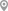JUDGES COURT RD, KolkataBediapara, KolkataJadavpur, KolkataAeepjay School, KolkataMalancha Mahinagar, KolkataPasupati Bhattacharjee Road, Kolkata

X

Post your Learning Need

Let us shortlist and give the best tutors and institutes.

or

Send Enquiry to Palak

Let Palak know you are interested in their class

Reply to 's review

Enter your reply*

1500/1500

Please enter your reply

Your reply should contain a minimum of 10 charactersYour reply has been successfully submitted.

UrbanPro.com is India's largest network of most trusted tutors and institutes. Over 55 lakh students rely on UrbanPro.com, to fulfill their learning requirements across 1,000+ categories. Using UrbanPro.com, parents, and students can compare multiple Tutors and Institutes and choose the one that best suits their requirements. More than 7.5 lakh verified Tutors and Institutes are helping millions of students every day and growing their tutoring business on UrbanPro.com. Whether you are looking for a tutor to learn mathematics, a German language trainer to brush up your German language skills or an institute to upgrade your IT skills, we have got the best selection of Tutors and Training Institutes for you. Read more